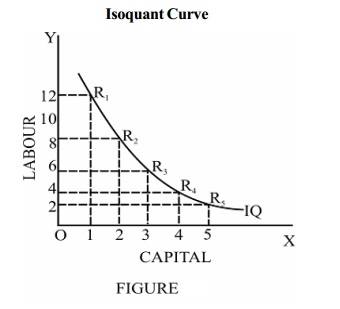Home | | Economics | Characteristics of an isoquant

# Characteristics of an isoquantThe isoquant analysis helps to understand how different combinations of two or more factors are used to produce a given level of output.

Production function through Iso-quants

The isoquant analysis helps to understand how different combinations of two or more factors are used to produce a given level of output. Considering two factors of production, (capital and labour) the following table shows various combinations of capital and labour that help a firm to produce 1000 units of a product.

Production with two variable inputs

Combination  Units of capital   Units of Labour  Output in units

A 1        12      1000

B 2        8        1000

C 3        5        1000

D 4        3        1000

E 5        2        1000From the above table, it is clear that all combinations with different quantities of labour and capital result in the same level of production of 1000 units.

The two axes measure the quantities of labour and capital and the curve IQ shows the different combinations that produce 1000 units of output. Each of the points R1, R2, R3, R4 and R5 on the curve shows a capital-labour combination that can produce 1000 units of output. Therefore the curve is known as an equal product curve or an iso-quant curve.

Thus an isoquant or isoproduct curve represents different combinations of two factors of production that yield the same level of output.

Characteristics of an isoquant

1.        The isoquant is downward sloping from left to right i.e. it is negatively sloped.

2.        An isoquant is convex to the origin because of the diminishing marginal rate of technical substitution. Marginal rate of technical substitution of factor X (capital) for factor Y (labour) may be defined as the amount of factor Y (labour) which can be replaced by one unit of factor X (capital), the level of output remaining unchanged.

MRTS C L =  ∆L/ ∆C

MRTS can be calculated using the above formula

Combination  Units of capital    Units of Labour    MRTS CL

A 1        12

B 2        8        4

C 3        5        3

D 4        3        2

E 5        2        1Thus the marginal rate of technical substitution is always declining. Hence the isoquant is always convex to the origin. The slope of the isoquant represents marginal rate of technical substitution.

3.        Higher the isoquant, higher will be the level of output produced. A set of isoquants which represents different levels of output is called 'isoquant map'. In the isoquant map, the isoquants on the right side represent higher levels of output and vice versa.

Isocost Line

The isocost line plays an important role in determining the combination of factors that the firm will choose for production. An isocost line is defined as locus of points representing various combinations of two factors, which the firm can buy with a given outlay. Higher isocost lines represent higher outlays (total cost) and lower isocost lines represent lower outlays.

The isocost line depends on two things :

4.        Prices of the factors of production and

5.        the total outlay, which a firm has to make on the factors of production. Given these two, the isocost line can be drawn. The slope of the isocost line is equal to the ratio of the prices of two factors. Thus the slope of the isocost line is given as

6.        Slope of isocost line = Price of factor X (Capital) / Price of factor Y (Labour)

Study Material, Lecturing Notes, Assignment, Reference, Wiki description explanation, brief detail
11th 12th std standard Indian Economy Economic status Higher secondary school College : Characteristics of an isoquant |